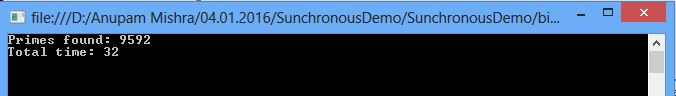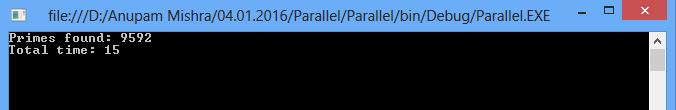##### Introduction:

We are working on multiprocessor for many years, they are available in almost every device. However, many developers are creating single-threaded programs, they are not taking advantages of all the extra processing power. Suppose you have many task to perform and many people to work on task but you are giving work to only one, it’s inefficient.

##### Parallel Programming?

Before explaining about parallel programming in c#, let me explain two concepts closely related to parallel programming i.e. synchronous and asynchronous execution modes that can effect performance of your task. When you execute a program synchronously, the program runs all tasks in sequence and then wait until it finishes before starting the next one while when it is executed asynchronously, the program does not run all tasks in sequence: it fires the tasks, and then waits for its completion.

If asynchronous execution takes less time in comparison to synchronous execution, so why we are using synchronous execution? In synchronous execution every task is executed in sequence. That’s a formal way which we are generally used.

Asynchronous execution eliminates disadvantages of synchronous modes: it is eliminate hang-up UI problem (because it can run as a background task in asynchronous execution), and it uses all cores of your processor for better performance. So, do you choose synchronous programming or better use of shared resources? So, don’t need to make decision. Microsoft has created several ways to minimize the difficulties of programming for asynchronous execution.

##### Asynchronous Programming models in .NET

In 2001, version 1.0 the Asynchronous Programming Model (APM) is introduced by Microsoft, but it’s complicated to implement. However, in .NET 2.0, Microsoft introduced a new model: the Event-Based Asynchronous Pattern (EAP).  EAP simplified things, but it was not enough, I do not discuss these models .So, in 4.0, Microsoft introduced Task Parallel Library (TPL) as a new model.

The Task Parallel Library (TPL):

The TPL is a major improvement over the previous models such as APM, EAP etc. It is better simplifies of parallel processing and makes better use of system resources. If you want to use parallel processing in your programs, TPL is the way to accomplishment of parallel programming in c#. For example, I will create a one synchronous program that find the prime numbers 2 to 10000. The program will be given an output how many prime number is found and total time to taken: and same process for the Asynchronous pattern.

##### Synchronous:
```using System;using System.Collections.Generic;using System.Diagnostics;using System.Linq;namespace SynchronousDemo{    class Program    {        static void Main(string[] args)        {            var sw = new Stopwatch();            sw.Start();            var primes = GetPrimeNumbers(2, 10000);            Console.WriteLine("Primes
found: {0}\nTotal time: {1}",
primes.Count, sw.ElapsedMilliseconds);            Console.ReadKey();        }         private static List<int> GetPrimeNumbers(int minimum, int maximum)        {            var count = maximum - minimum + 1;            return Enumerable.Range(minimum, count).Where(IsPrimeNumber).ToList();        }         static bool IsPrimeNumber(int p)        {            if (p % 2 == 0)                return p == 2;            var topLimit = (int)Math.Sqrt(p);            for (int i = 3; i <= topLimit; i += 2)            {                if (p % i == 0) return false;            }            return true;        }    }}```

##### Output:Asynchronous: The TPL you can create implicitly or explicitly of task. To create a task implicitly, you can use the Parallel class—a static class that has the three methods:  For, ForEach, and Invoke methods. For and ForEach allow loops to run task in parallel;

Invoke allows to several operation to create queue in parallel.

```using System;using System.Collections.Generic;using System.Diagnostics;using System.Linq;using System.Threading.Tasks; namespace ParallelDEmo{    class Program    {        static void Main(string[] args)        {            var sw = new Stopwatch();            sw.Start();            const int numThreads = 10;            var primes = new List<int>[numThreads];            Parallel.For(0, numThreads, i => primes[i] =                         GetPrimeNumbers(i
== 0 ? 2 : i * 10000 + 1, (i + 1)* 10000));            sw.Stop();            Console.WriteLine("Primes
found: {0}\nTotal time: {1}",
primes.Sum(p => p.Count),        sw.ElapsedMilliseconds);                 Console.ReadKey();        }         private static List<int> GetPrimeNumbers(int minimum, int maximum)        {               var count = maximum - minimum + 1;               return Enumerable.Range(minimum, count).Where(IsPrimeNumber).ToList();        }         static bool IsPrimeNumber(int p)        {            if (p % 2 == 0)                  return p == 2;            var topLimit = (int)Math.Sqrt(p);            for (int i = 3; i <= topLimit; i += 2)            {                if (p % i == 0) return false;            }                 return true;        }    }}```

##### Output:##### Parallel Linq(PLINQ)

Parallel Linq (PLINQ) is stands parallel implementation for the LINQ query language. Parallel LINQ (PLINQ) is a parallel implementation of the LINQ pattern. You can easily transform LINQ queries to parallel by using AsParallel method.

##### Async Programming

In version 5.0, Microsoft introduced two new keywords async and await. Both are the heart of async programming. By using those two keywords, you can use resources in the .NET Framework or the Windows Runtime to create an asynchronous method almost as easily as you create a synchronous method. Asynchronous methods that you define by using async and await are referred to as async methods.

For more details, you see also:

Modified On Jul-04-2019 10:49:44 AM